Go back to  'Functions'

We have had a basic discussion on how to graph quadratic expressions, in the chapter on quadratic equations. Now, in terms of graph transformations, we will understand a step-by-step procedure to plot the graph of any quadratic function.

$f\left( x \right) = a{x^2} + bx + c$

First, we rearrange it (by the method of completion of squares) to the following form:

$f\left( x \right) = a{\left( {x + \frac{b}{{2a}}}\right)^2} - \frac{D}{{4a}}$

The term D is the discriminant, given by $$D = {b^2} - 4ac$$. If you are unsure about this rearrangement, you are urged to refer to the chapter on quadratic equations.

Now, to plot the graph of f,we start by taking the graph of $${x^2}$$ ,and applying a series of transformations on it:

Step-1: $${x^2} \to a{x^2}$$: This will imply a vertical scaling of the original parabola. If a is negative, the parabola will also flip its mouth from the positive to the negative side. The magnitude of the scaling depends upon the magnitude of a.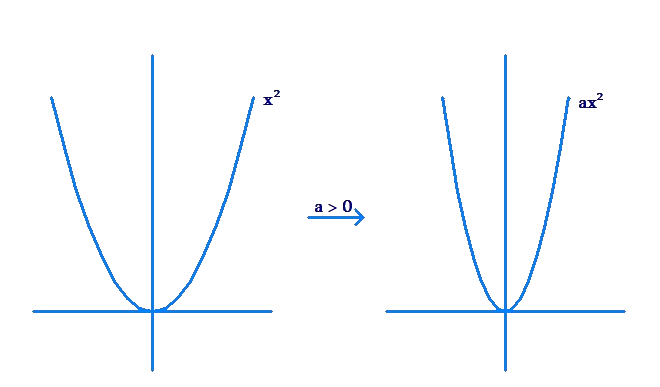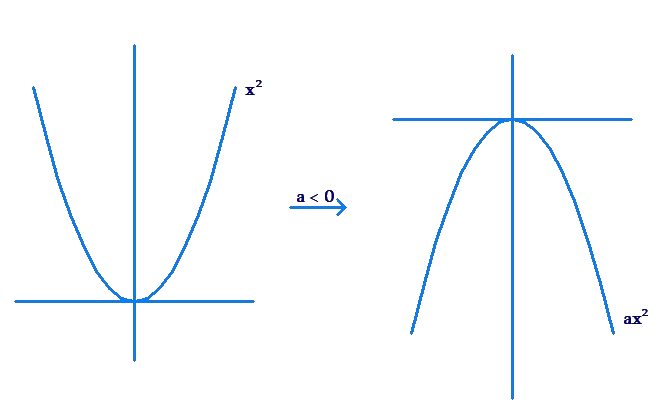Step-2:  $$a{x^2} \to a{\left( {x + \frac{b}{{2a}}}\right)^2}$$ : This is a horizontal shift of magnitude $$\left| {\frac{b}{{2a}}} \right|$$ units. The direction of the shift will be decided by the sign of $$\frac{b}{{2a}}$$. The new vertex of the parabola will be at $$\left( { -\frac{b}{{2a}},0} \right)$$. The following figure shows an example shift: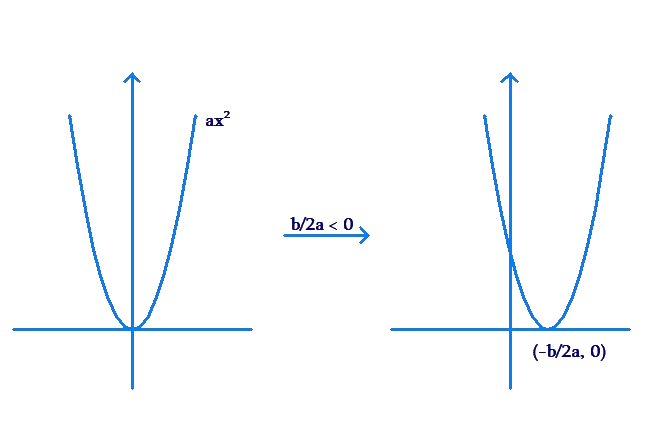Step-3: $$a{\left( {x + \frac{b}{{2a}}} \right)^2} \to a{\left( {x + \frac{b}{{2a}}} \right)^2} - \frac{D}{{4a}}$$: This transformation is a vertical shift of magnitude $$\left| {\frac{D}{{4a}}} \right|$$ units. The direction of the shift will be decided by the sign of $$\frac{D}{{4a}}$$. The final vertex of the parabola will be at $$\left( { - \frac{b}{{2a}},- \frac{D}{{4a}}} \right)$$. The following figure shows an example shift: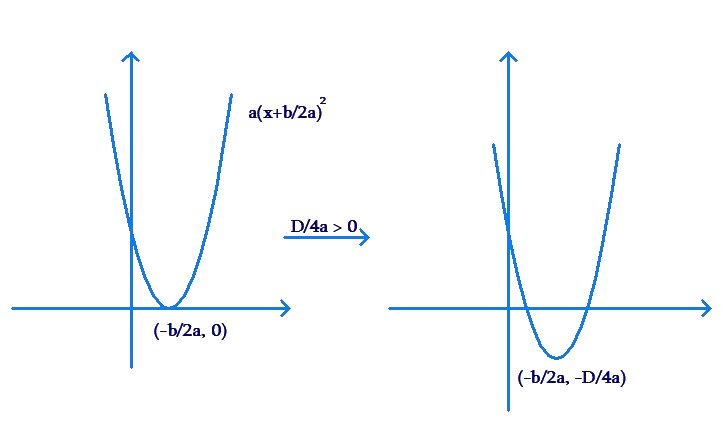To summarize:

1. If a is positive, the parabola will open upward, else it will open downward.

2. The coordinates of the vertex of the parabola are $$\left({ - \frac{b}{{2a}}, - \frac{D}{{4a}}} \right)$$.

Example 1: Plot the graph of $$f\left( x \right) = 1 - 2x -3{x^2}$$.

Solution: We have:

$\begin{array}{l}a = - 3,\,\,\,b = - 2,\,\,\,c = 1\\ \Rightarrow \,\,\,D = {b^2} - 4ac =16\end{array}$

The coordinates of the vertex are.

$V \equiv \left( { - \frac{b}{{2a}}, -\frac{D}{{4a}}} \right) = \left( { - \frac{1}{3},\frac{4}{3}} \right)$

Note that the parabola will open downward (since a is negative), but the vertex has a positive y-coordinate.This means that the parabola crosses the x-axis. In other words, the quadratic function as real zeroes. Let us calculate these zeroes:

$x = \frac{{ - \left( { - 2} \right) \pm \sqrt {16}}}{{ - 6}} = - 1,\frac{1}{3}$

The parabola will cross the x-axis at these x values.

Finally, using all this information,we plot the graph: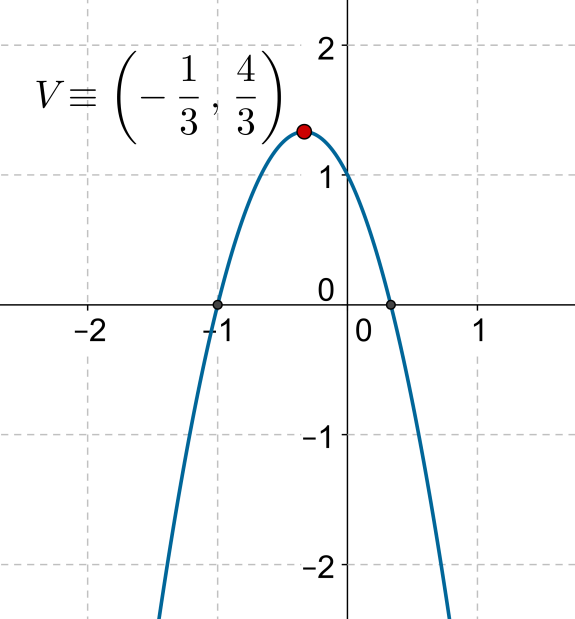Example 2: Plot the graph of $$f\left( x \right) = 5x - 4 -{x^2}$$.

Solution: We have:

$\begin{array}{l}a = - 1,\,\,\,b = 5,\,\,\,c = - 4\\ \Rightarrow \,\,\,D = {b^2} - 4ac =9\end{array}$

Since a is negative, the parabola will open downward. The coordinates of the vertex are

$V \equiv \left( { - \frac{b}{{2a}}, -\frac{D}{{4a}}} \right) = \left( {\frac{5}{2},\frac{9}{4}} \right)$

We can calculate the roots using the quadratic formula or through factorization; we use the latter approach in this case:

$\begin{array}{l}f\left( x \right) = 5x - 4 - {x^2} =0\\ \Rightarrow \,\,\,{x^2} - 5x + 4 =0\\ \Rightarrow \,\,\,\left( {x - 1}\right)\left( {x - 4} \right) = 0\\ \Rightarrow \,\,\,x = 1,4\end{array}$

Using this information, we plot the graph of  f: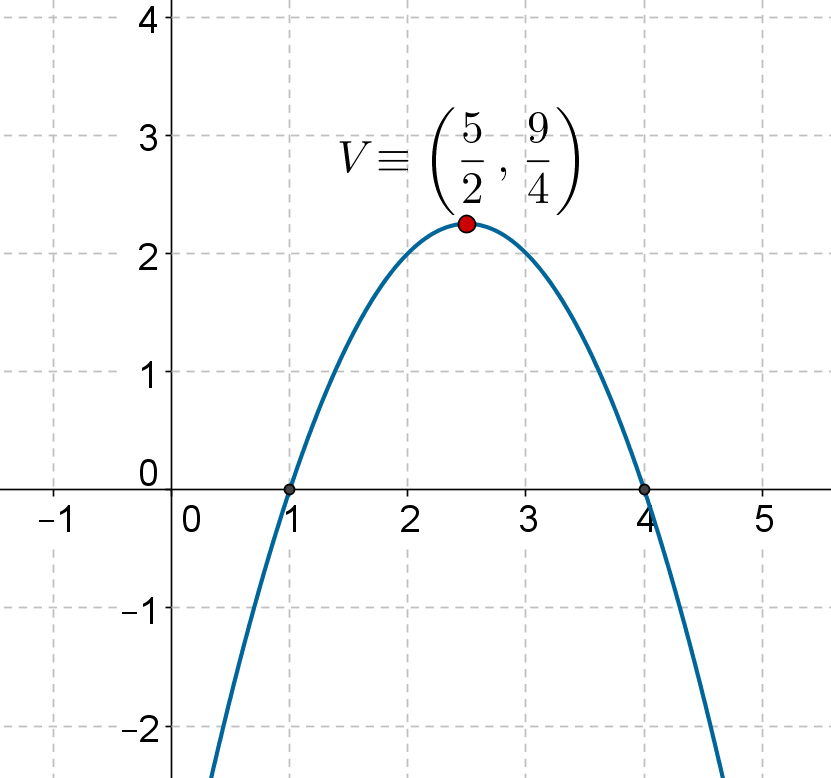Functions
Functions
grade 10 | Questions Set 2
Functions
Functions
grade 10 | Questions Set 1